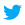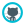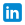dataewan

Markov Chain Monte Carlo (MCMC) sampling

Markov Chain Monte Carlo (MCMC) uses this description of Bayes rule

Where we say that the shape of the posterior distribution only depends on the likelihood (p(data | θ)), and the prior (p(θ)). We're able to generate samples from a distribution that has exactly the same shape as the posterior, but isn't normalised.

MCMC is a set of techniques that draw samples from the posterior probability distribution, using this property.

I'll demonstrate one type of MCMC called Metropolis sampling. There's a really good blog post called MCMC sampling for dummies, which demonstrates this technique using Python. I wrote out this Python code, and this gave me a good intuition of Metropolis sampling. It describes fitting a simple model to data: with 20 data points, what is the mean of the normal distribution that fits this data?

We start with a value for the model parameter. This value that we pick is arbitrary. We'll call this value $\mu_c$ .

2. Propose a new value

The next thing that we do is propose a new value for the model parameters. We do this by taking a sample from a normal distribution with mean value $\mu_c$ , and with a standard deviation of a parameter called step size.

step size is a parameter we can set. If we set this bigger then we'll take larger jumps, if we set it smaller then we make smaller jumps from one point to the next.

3. Calculate the likelihood and prior values for the current and proposed parameter

We want to calculate the nominator part of Bayes rule:

This is the likelihood multiplied by the prior. The likelihood is how likely it is for the data to be generated from a normal distribution with that mean. We get the probability for each datapoint and multiply them together.

# We're assuming that the standard deviation is fixed at 1,
# and only the mean changes.
likelihood_current = stats.norm(mu_current, 1).pdf(data).prod()
likelihood_proposal = stats.norm(mu_proposal, 1).pdf(data).prod()

The prior is how credible we thought that value for mean was before we looked at the data. We described our prior as a normal distribution that we parameterised.

prior_current = stats.norm(mu_prior_mu, mu_prior_sd).pdf(mu_current)
prior_proposal = stats.norm(mu_prior_mu, mu_prior_sd).pdf(mu_proposal)

4. Should we accept the new point?

We calculate the ratio between these two numerator terms for the proposed and current points. Call this r.

If r is greater than 1, that means that the new parameter is a better description than the previous one, and we always accept this point.

But we don't want to only accept better points. If we do that we get stuck at a maximum point of the distribution and don't explore the whole space. So if r is less than 1 we accept that point with probability equal to r.

We now loop round to step 1 again, but with our new value for μ, or our old one if we rejected the new point.